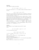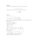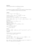# Exercise sheet 5 pdfLoading previews...asymptotic expansions as solutions of differential equationsasymptotic expansions as solutions of differential equationsasymptotic expansions as solutions of differential equationsasymptotic expansions as solutions of differential equationsasymptotic expansions as solutions of differential equations
11 files in this resource

## Exercise sheet 5 pdf

Exercises and solutions in PDFView Item